Starts With A Bang

# Why June 28th Is The Only ‘Perfect’ Day Of The Year

#### Whether you write it 6/28 or 28/6, it’s perfection either way.

Perfection might be a wonderful thing to strive for in life, but achieving it is very rare. In the realm of mathematics, however, perfection is even more difficult to find than it is in life. Despite all the numbers that we know exist — not only just from 1 to infinity, but far beyond — only a few of them can be considered “perfect numbers.” For most of human history, only a handful of perfect numbers were known, and even today — with the advent of modern mathematical techniques and all the computational advances that have occurred — we only know of 51 perfect numbers in total.

It just so happens that June 28th, or the 28th day of the 6th month of the year, is the only day/month combination that involves two mathematically perfect numbers: 6 and 28. The next “perfect” number doesn’t occur until 496, and you won’t find the fourth until you get all the way up to 8128. That means, if you follow our calendar, June 28, 496 was the first “perfect day” in history, and the next one won’t come until June 28, 8128.

Nevertheless, June 28 is the perfect day for a celebration of mathematical perfection. Here’s an explanation that everyone can follow.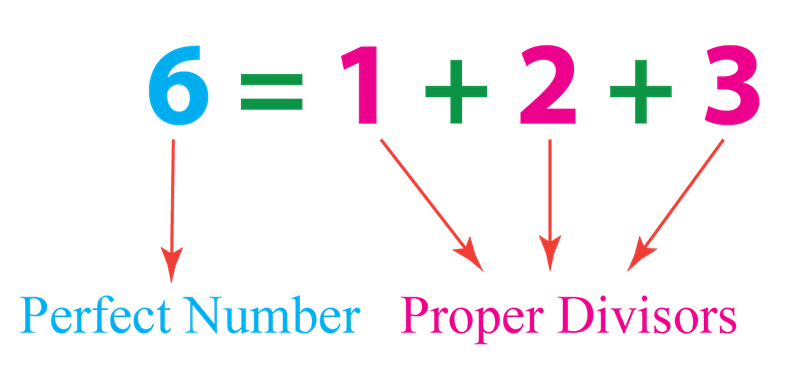The first mathematically perfect number, 6, with its proper divisors 1, 2, and 3. A number is perfect if the sum of all of its positive integer factors, excluding itself, adds up to the original number itself. In the case of 6, its factors 1, 2, and 3 do in fact sum up to 6. (YOGESHKUMAR HADIYA / C-SHARPCORNER.COM)

I want to introduce you, in a way you might not conventionally think about it, to the number 6. Unlike all the other numbers around it, 6 is not just special, but perfect.

What makes it perfect?

Every positive integer — that is, every number that you can imagine in the sequence 1, 2, 3, …, all the way up as high as you care to go — can be factored. Factoring a number means you can express it as two whole numbers multiplied together. Every number has, as two of its factors, itself and the number 1.

If you have no other factors besides 1 and the number itself, you’re a prime number.

If you do have other factors, however, you can add them all up. If, when you do that, the sum of all of your factors (excluding the original number) equals the original number itself, then congratulations: you are, in fact, a perfect number. And that’s exactly what happens for the number 6.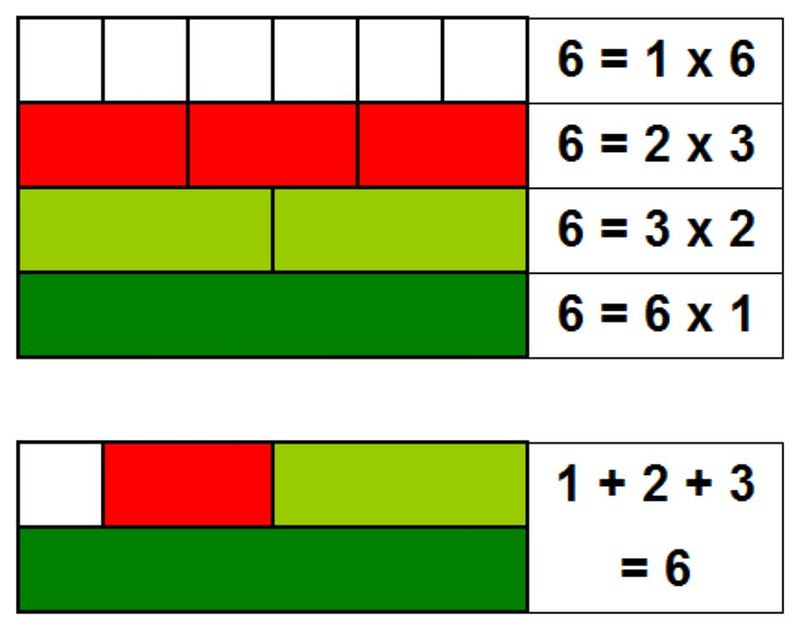The various ways to factor the number 6, illustrating its perfection. Six is a perfect number because all of its unique, positive integer factors excluding itself sum up to itself. 1 + 2 + 3 = 6, and hence, 6 is perfect. (HYACINTH / WIKIMEDIA COMMONS / CCA-SA-4.0)

We can write down 6 as the product of two whole numbers, multiplied together, in two different ways:

• 6 × 1 = 6,
• 3 × 2 = 6,

and that’s it. All together, the factors of 6 are: 1, 2, 3, and the original number itself, 6. If you add up all of those factors — remember, excluding the original number itself — you can see that you get the original number back: 1 + 2 + 3 = 6.

That’s what makes a number “perfect.”

What if you’re not perfect? If the sum of all of your factors (except the original number) is less than the original number, you’re known as “deficient” instead. The idea that something would be a “perfect 10” is a mathematical travesty, as the factors of 10, other than itself, are: 1, 2, and 5. They only add up to 8, making 10 a deficient number.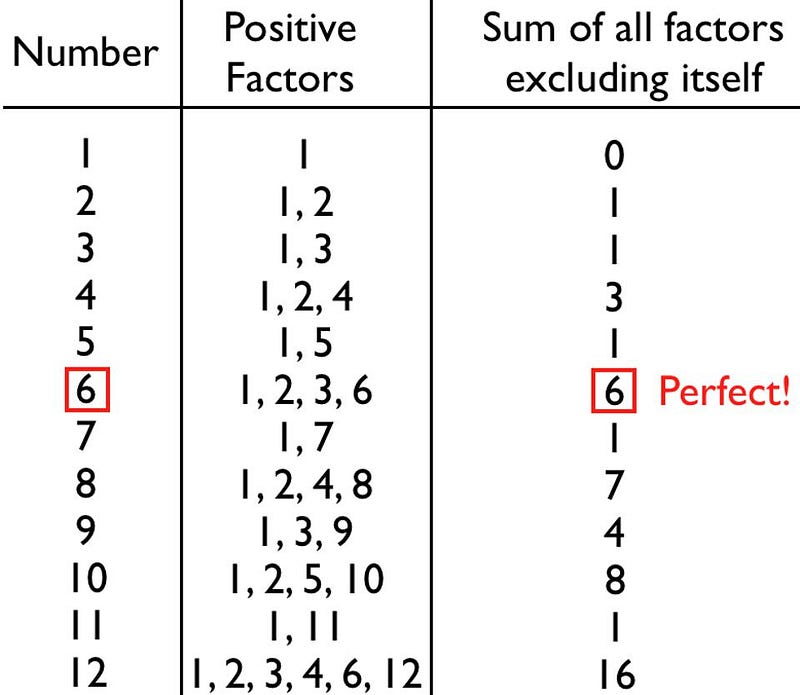The first few countable numbers are mostly deficient, but 6 is a perfect number: the first and easiest one to discover. Meanwhile, 12 is the first abundant number, while the one number often used to describe something that’s ‘perfect,’ 10, is actually itself deficient. (E. SIEGEL)

On the other hand, the sum of your factors (except the original number) could be greater than the original number, which would make you “abundant” instead. 12, for example, is an abundant number, since you can factor it as:

12 × 1 = 12,

6 × 2 = 12,

or 4 × 3 = 12.

The factors of 12, then, excluding itself, are: 1, 2, 3, 4, and 6, which adds up to 16, rendering 12 an abundant number.

Most numbers are deficient, and the overwhelming remainder are abundant. Only a very, very select few are perfect. In fact, if you could exhaustively try all the numbers, in order, to see whether they were deficient, abundant, or perfect. As you went up from 1, you’d find that every number was deficient until you got to 6, the first perfect number, and then you’d find that every other number was deficient except for 12, 18, 20, and 24 which are all abundant. At last, when you reached 28, you’d find another number that was neither deficient nor abundant; you’d find the second perfect number.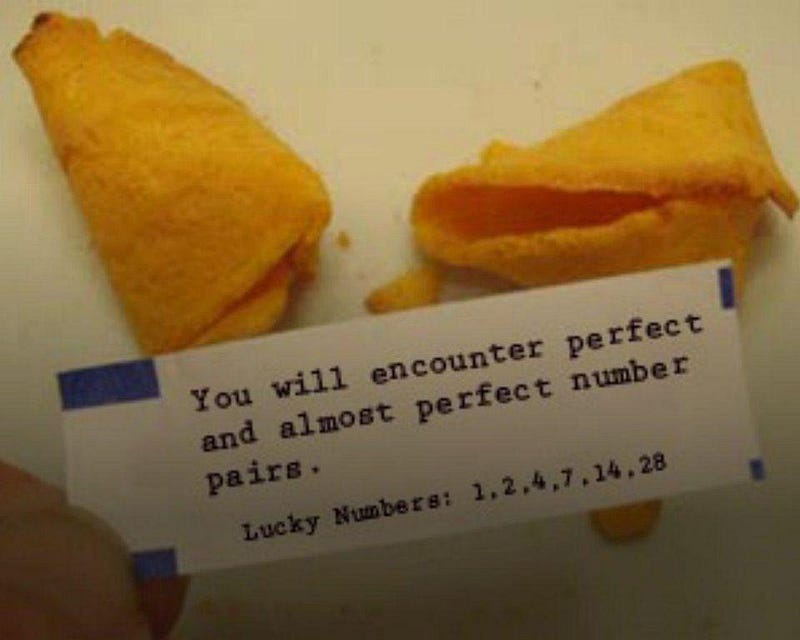While it might seem that calling a number ‘perfect’ is subjective, it has a mathematical definition that only a few numbers meet. The second one, 28, comes about because the factors of 28 smaller than itself are: 1, 2, 4, 7, and 14, which sum up to 28 itself. (JUDD SCHORR / GEEKDAD)

Why is 28 perfect? Because of its factors:

28 × 1 = 28,

14 × 2 = 28,

and 7 × 4 = 28.

As you can see, 1 + 2 + 4 + 7 + 14 = 28, making 28 the second perfect number. It’s pretty difficult to see if there’s a pattern to these perfect numbers with only the first two of them, so let’s take a look at the third as well: 496.

496 is also perfect, as its factors come from:

496 × 1 = 28,

248 × 2 = 496,

124 × 4 = 496,

62 × 8 = 496,

and 31 × 16 = 496.

And, just to check, you can verify that 1 + 2 + 4 + 8 + 16 + 31 + 62 + 124 + 248 does, in fact, sum up to 496.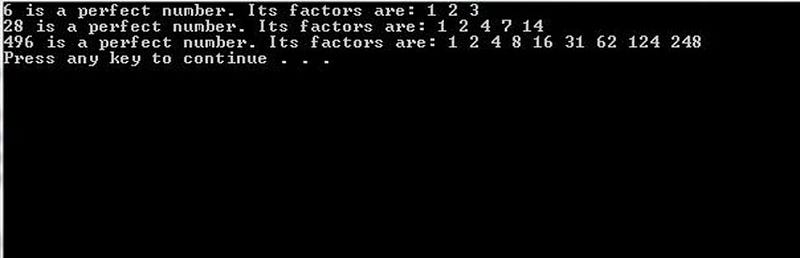Computer programs with enough computational power behind them can brute-force analyze a candidate Mersenne prime to see if it corresponds to a perfect number or not, using algorithms that run without flaw on a conventional (non-quantum) computer. For small numbers, this can be accomplished easily; for large numbers, this task is extremely difficult and requires ever more computational power. (C++ PROGRAM ORIGINALLY FROM PROGANSWER.COM)

Take a look (again, if you need to) at the various ways to factor these three perfect numbers: 6, 28, and 496.

Do you notice that the smaller “factor” in each of the ways to make these numbers follows a pattern?

• For 6, the smaller numbers are 1 and 2 in the two ways to factor 6.
• For 28, the smaller numbers are 1, 2, and 4 in the three ways to factor 28.
• For 496, the smaller numbers are 1, 2, 4, 8, and 16 in the five ways to factor 496.

Look at both the number of ways to factor the first three perfect numbers, as well as the small number in each of those multiplicative examples.

• 6: two ways to factor, and the sequence goes: 1, 2.
• 28: three ways to factor, and the sequence goes: 1, 2, 4.
• 496: five ways to factor, and the sequence goes: 1, 2, 4, 8, 16.

Even if you didn’t know what the fourth perfect number would be — and spoiler, it’s 8128 — how would you guess this pattern continues?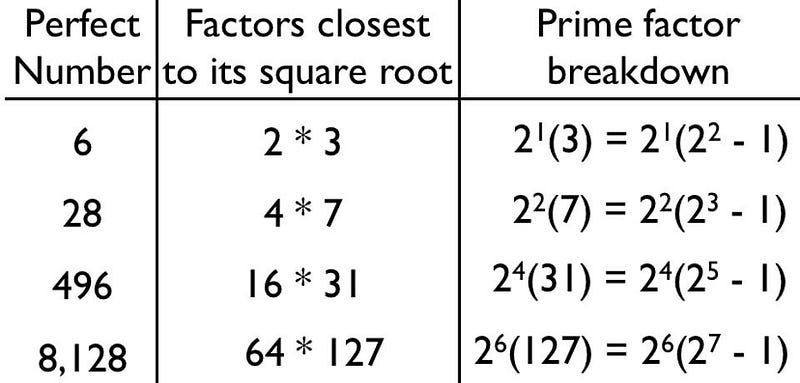The first four perfect numbers can be broken down by pulling out factors of 2 until you can no longer do so. Once that is achieved, you’re left with an odd number multiplied by ‘powers of 2’, where that odd number is 1 less than a power of 2 itself. If that odd number is prime, then this will generate a perfect number for you. (E. SIEGEL)

Congratulations are in order if you guessed that, for the fourth perfect number, you’d expect there were seven ways to factor it, and the sequence of the small number in each of the examples would go: 1, 2, 4, 8, 16, 32, and 64.

Why should you have guessed that?

Because the number of ways to factor something is following a pattern: 2, 3, 5, etc., all appear to be prime numbers. The next prime after 5 is 7, followed by 11, and then followed by 13, 17, 19, and so on. Meanwhile, the sequence of the smaller number in the various ways to factor the larger number appears to be following “powers of two.” For example, the five ways to factor 496 include 1, 2, 4, 8, and 16, which is equivalent to 2⁰, 2¹, 2², 2³, and 2⁴.

Well, how well does this mathematical intuition bear out in reality?

For the fourth perfect number, 8128, it holds up perfectly:

8128 × 1 = 8128,

4064 × 2 = 8128,

2032 × 4 = 8128,

1016 × 8 = 8128,

508 × 16 = 8128,

254 × 32 = 8128,

and 127 × 64 = 8128.

When you add these (non-self) factors up, again, 1 + 2 + 4 + 8 + 16 + 32 + 64 + 127 + 254 + 508 + 1016 + 2032 + 4064 checks out, as it really does equal 8128.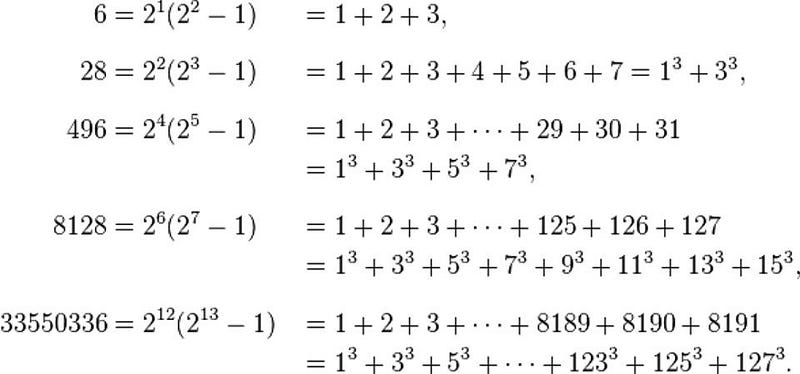The first five perfect numbers, where the one you might expect to be fifth, 2096128, does not appear. There are many interesting numerical properties surrounding perfect numbers, but they are not as easy to ‘guess’ from previous patterns as you might naively expect. (WIKIPEDIA PAGE ON PERFECT NUMBERS)

At this point, you’re probably thinking that you can take any prime number (and “generate” a perfect number from it by following this pattern. After all, the first four primes corresponded to the first four perfect numbers: 2, 3, 5, and 7 correspond to 6, 28, 496, and 8128. Mathematically, there’s a nice, compact way to write this correspondence by using the last factoring example in each of these cases:

6 = 2 × 3 = 2¹ × (2²–1),

28 = 4 × 7 = 2² × (2³–1),

496 = 16 × 31 = 2⁴ × (2⁵–1),

and 8128 = 64 × 127 = 2⁶ × (2⁷–1).

But when we come to the next prime — 11 — we see a spectacular breakdown. You’d fully expect, following the same pattern, that 2¹⁰ × (2¹¹–1) would be a perfect number. When you work it out, that should be 1024 × 2047, which equals 2096128. Which, if you check for yourself, is not a perfect number.

Why not? For each of the prior four examples, the one and only “odd” factor that they possess — 3, 7, 31, and 127, respectively — is also prime. But in the case of this attempted fifth example, 2047 is not prime, but can be factored: 2047 = 23 × 89. Instead of perfect, 2096128 turns out to be an abundant number. (Today, we know that a little under 25% of all positive integers are abundant, a little over 75% are deficient, and that perfect numbers are extraordinary rarities.)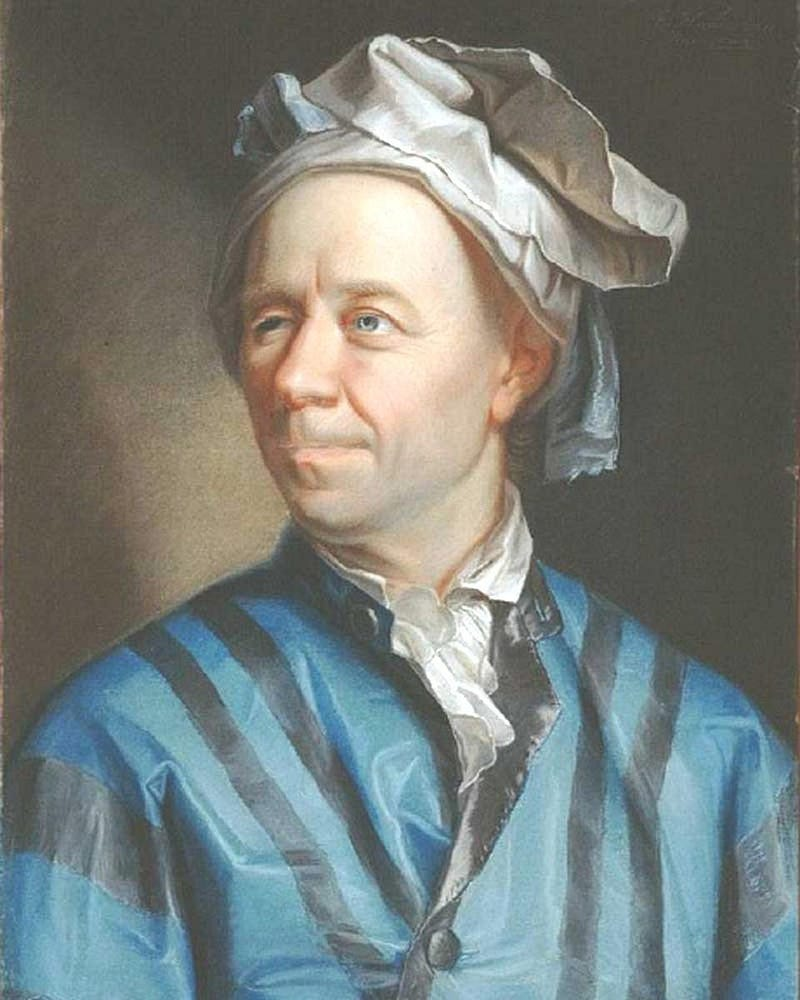Leonhard Euler, famed mathematician, discovered the Mersenne Prime 2³¹-1, which corresponds to a perfect number. Discovered in 1772 by Euler, it remained the largest known prime for over 90 years. There is an unproven conjecture that 2²¹⁴⁷⁴⁸³⁶⁴⁷–1 is a Mersenne Prime, too. (JAKOB EMANUEL HANDMANN, PAINTER)

What this teaches us is that we have a simple way for generating perfect number candidates, but then we have an extra step to do: check whether one specific number — the one factor remaining when all the powers of 2 are pulled out of the perfect number candidate — is prime.

The ones that successfully generate perfect numbers fall into a special category all their own: the Mersenne primes. As of 100 years ago, there were only 12 Mersenne primes (and hence, only 12 perfect numbers) known. One spectacular advance came in 1903, when Frank Nelson Cole gave a talk to the American Mathematical Society entitled “On the Factorization of Large Numbers.” On the left side of the board, he calculated (2⁶⁷–1), obtaining 147,573,952,589,676,412,927. On the right side, he simply wrote: 193,707,721 × 761,838,257,287. He spent the next hour performing the multiplication of these two numbers by hand, saying no words until the answer was reached: 147,573,952,589,676,412,927.Travel the Universe with astrophysicist Ethan Siegel. Subscribers will get the newsletter every Saturday. All aboard!

According to legend, he took his seat and immediately received a standing ovation: the first one ever given at a mathematics talk. (Today, that calculation can be performed in seconds by a typical computer.)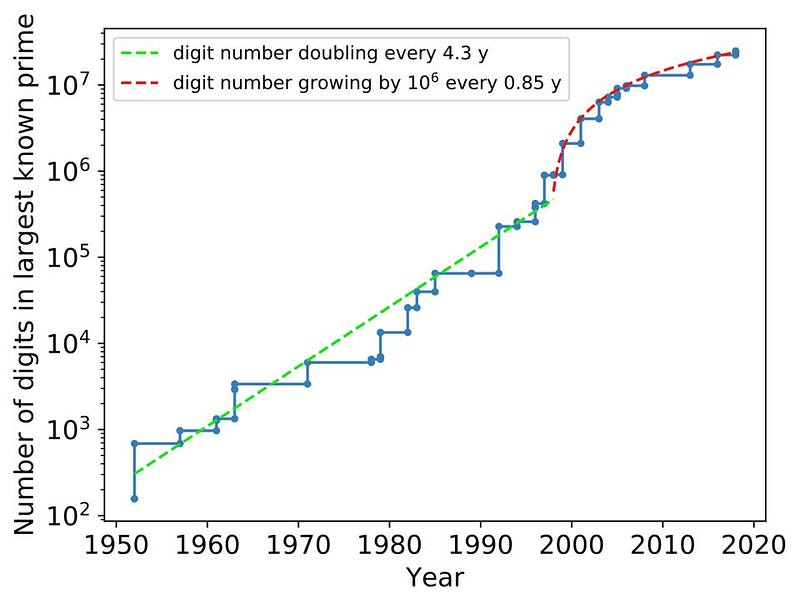This logarithmic plot shows the number of digits in the largest Mersenne prime vs. time. Prior to 1952, only 12 Mersenne primes were known. With the advent of computers, however, as well as novel algorithms, the number of digits in the largest known Mersenne prime has grown exponentially, with the advent of GIMPS causing it to grow even faster since 1997. (NICOGUARO / WIKIMEDIA COMMONS / CCA-SA-4.0)

As of 2021, there are 51 known Mersenne primes, with every discovery since late 1996 achieved as a part of the Great Internet Mersenne Prime Search. The largest one, as of Perfect Number Day in 2021, is 2⁸²⁵⁸⁹⁹³³–1, which creates a perfect number (when multipled by 2⁸²⁵⁸⁹⁹³²) with nearly 50,000,000 digits to it. If you can find (and verify) a Mersenne prime with 100,000,000 digits or more, you’ll win a cash prize of \$150,000 dollars, and if you can find (and verify) one with a billion digits, that prize rises up to \$250,000.

If you’re ambitious and have a lot of time and computing power at your disposal, I even have an interesting candidate for you to examine: (2²¹⁴⁷⁴⁸³⁶⁴⁷–1), where 2147483647 itself is the eight Mersenne prime: (2³¹–1). With about 600 million digits to it, it would be the largest Mersenne prime ever verified. (That is, if it turns out to be prime.)

But for numbers with one or two digits, only two of them are perfect: 6 and 28. Whether you write the month or date first, that makes June 28 the only “perfect” day of the year, a mathematical fact you can enjoy — and, if you like, explore — any time you like!

Starts With A Bang is written by Ethan Siegel, Ph.D., author of Beyond The Galaxy, and Treknology: The Science of Star Trek from Tricorders to Warp Drive.

Related
Who — or what — really controls your mind?
More than a century ago, Halifax suffered an accidental blast one-fifth the size of the atomic bomb dropped on Hiroshima.
Dive into the twisted truths and concealed realities told by literature's most unreliable narrators.
If you don’t feel better after the weekend, the “burnout paradox” could explain why.
7 min
with
If you can identify a foreground star, the spike patterns are a dead giveaway as to whether it's a JWST image or any other observatory.

Up Next
A new study proposes that Hawking radiation could be used to find dark matter in places like primordial black holes.